# Linear Regression on Boston House Pricing dataset using python

1. Introduction:
1. There should be a Linear and Additive relationship between dependent (output: y) and independent (inputs: X’s) variables. The linear relationship creates a straight line when plotted on a graph. An additive relationship means that the effect of X’s on y is independent of other variables.
2. There should be no correlation between the error or residual terms. The absence of this phenomenon is known as Autocorrelation.
3. The independent (X’s) variables should not be correlated, the absence of phenomena is called multi-collinearity.
4. The error term must have constant variance. The phenomenon is known as homoskedasticity. The presence of non-constant variance is referred to as heteroskedasticity.
5. The error term must be normally distributed.
• data: contains the information for various houses
• target: prices of the house
• feature_names: names of the features
• DESCR: describes the dataset

--

--

--

## More from Heena Sharma

Love podcasts or audiobooks? Learn on the go with our new app.

## Dive head first into advanced GANs: exploring self-attention and spectral norm## ML Algorithms: One SD (σ)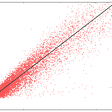## Heating up word2vec: BlazingText for Real-time Search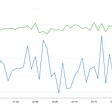## Dependency Parser or how to find syntactic neighbours of a word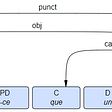## An Introduction to Gradient Descent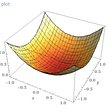## Machine Learning With SecOps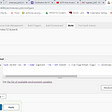## Precision and recall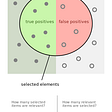## An illustrative introduction to Fisher’s Linear Discriminant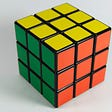## Linear Regression in Machine Learning: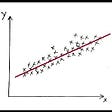## Simple Linear Regression Using Example.## Linear Regression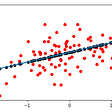## Example Study Cases with Random Forest Regression